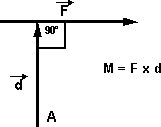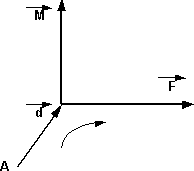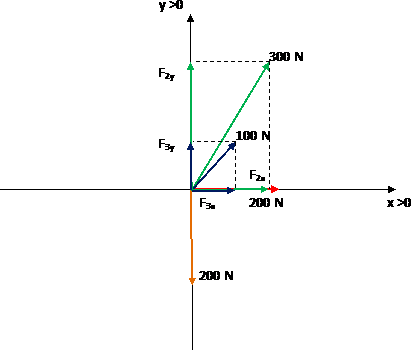Static

# Static

Vectors : They are mathematical models.

Let be the vector V , it represents a physical quantity, it is composed of:

1) Modulus : (magnitude) numerical and absolute value of the same, expresses the quantity that it represents and is assigned a unit

2) Direction : line of action, which according to the reference system has an inclination α

3) Direction : depending on the reference system, it will have a positive or negative sign

4) Origin : application point## Force

Physical magnitude that is represented by a vector and its unit can be newton ( N ), kilogram force (kg) or dyne (dyn).

### Resulting

It is the vector sum of all applied and unapplied forces on a system.

## moment of a force

The moment of a force is the product of said force by the perpendicular distance to a determined axis of rotation. When a force is applied to a heavy door to open it, the force is exerted perpendicular to the door and at its maximum distance from the hinges. Thus a maximum moment is achieved. If the door were pushed with the same force at a point midway between the handle and the hinges, the magnitude of the moment would be halved. If the force were applied parallel to the door (ie edgewise), the moment would be zero.Let the distance vector be a vector perpendicular to a force, of magnitude equal to the distance between a point a and the line of action of the force, it is defined as the moment vector of the force with respect to point a:

 Clockwise – FCounterclockwise + FThe vector product between the force vector and the distance vector, whose direction is perpendicular to the plane formed by point A and the force, and the direction will depend on the force vector (clockwise – counterclockwise).Three-dimensional view according to the corkscrew rule (for the left hand)

The units of the moment vector are: N m, kilogram (kgm) or dyn cm as this is a vector product.

## See example no. 1

Yes:

1 = 200 N, on the X axis directed to the right.

2 = 300 N, 60° above the X axis , to the right.

3 = 100 N, 45° about the X axis , to the right.

4 = 200 N, in the negative direction of the Y axis .

Find the resultant force.

### Solution

We graph the forces and then project the components onto the axes:Then we add the components on each axis:

In X :

1 + F 2x + F 3x = F Tx (total force at x)

In AND :

2y + F 3y – F 4 = F Ty (total force in y)

To find the value of each component we use trigonometry, cosine and sine.

 cos 60° = F2x _ 300N

F2x = 300 N cos 60° ⇒ F2x = 300 N 0.5 ⇒ F2x = 150 N

 cos 45° = f 3x 100N

F3x = 100 N cos 45° ⇒ F3x = 100 N 0.71 ⇒ F3x = 70.71 N

 sin 60° = F2y _ 300N

F2y = 300 N sin 60° ⇒ F2y = 300 N 0.87 ⇒ F2y = 259.81 N

 sin 45° = F3y _ 100N

F3y = 100 N sin 45° ⇒ F3y = 100 N 0.71 ⇒ F3y = 70.71 N

Finally we put together the equations and do the math:

In X :

200 N + 150 N + 70.71 N = FTx ⇒ FTx = 420.71 N

In AND :

259.81 N + 70.71 N – 200 N = FTy ⇒ FTy = 130.52 N

We already have the components of the resultant force:

FT x = 420.71 N

Ty = 130.52 N

Now we have to find the module of the compound force using the Pythagorean theorem:

FT ² = FTx ² + FT Ty ² ⇒ FT ² = ( 420.71 N)² + (130.52 N) ²

FT² = 17,035.4704 N² + 176,996.9041 N² ⇒ FT² = 194,032.3745 N² ⇒ FT = 440.49 N

Again with trigonometry we find the angle, where α is the angle between the force and the X axis :

 tg α = F Ty FTX _
 artg F Ty = α FTX _
 α = arctg 130.52N 420.71N

α = arctg 0.31 ⇒ α = 17.24°

Result, the module of the resultant force is:

FT = 440.49 N

Result, the á of the resultant force is:

α = 17.24°